﻿ 基于MIMO异构Ad Hoc网络节点调度优化算法 Node Scheduling Optimization Algorithm in Heterogeneous Ad Hoc Networks Based on MIMO

Hans Journal of Wireless Communications
Vol.07 No.05(2017), Article ID:22679,8 pages
10.12677/HJWC.2017.75019

Node Scheduling Optimization Algorithm in Heterogeneous Ad Hoc Networks Based on MIMO

Wanmei Feng

School of Physics and Telecommunication Engineering, South China Normal University, Guangzhou Guangdong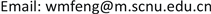Received: Oct. 24th, 2017; accepted: Nov. 7th, 2017; published: Nov. 15th, 2017ABSTRACT

Multiple-input and multiple-output (MIMO) can obviously improve transmission reliability and bandwidth efficiency in wireless networks. The nodes in heterogeneous Ad Hoc networks enable to communicate directly without wired connections, making up the lack of communication with fixed network infrastructures. Therefore, heterogeneous Ad Hoc networks combined with MIMO techniques becomes one of the research hotspots in academia. However, in heterogeneous Ad Hoc networks based on MIMO, different transmitter-receiver pairs transmit data streams by different links which have the effect of co-channel interference. A good scheduling algorithm plays an important part in reducing the co-channel interference. According to the characteristics of heterogeneous Ad Hoc networks and the contention regions between links, we propose node scheduling optimization algorithm to obtain resource allocation (rate). Based on different rate of different links, transmitters can choose the optimum receivers to transmit data streams via node scheduling. The results of the experience proof that node scheduling optimization algorithm can highly improve the system throughput with reducing the effect of co-channel interference.

Keywords:MIMO, Heterogeneous Ad Hoc Network, Node Scheduling, Interference1. 引言

2. 基本原理

2.1. 信道模型

${h}_{pq}=\sqrt{\frac{k}{k+1}}\text{ }{\sigma }_{\iota }{\text{e}}^{j\theta }+\sqrt{\frac{1}{k+1}}\text{ }\mathcal{C}\mathcal{N}\left(0,{\sigma }_{ı}^{2}\right)$ (1)

𝒷(s) $=\mathrm{log}\left(1+{p}_{s}{h}_{s}^{\text{*}}{\left({N}_{0}{I}_{{N}_{d\left(s\right)}^{ant}}+{\sum }_{q\in I\left(s\right)}{p}_{q}{h}_{q}{h}_{q}^{\text{*}}\right)}^{-1}{h}_{s}\right)$ (2)

${p}_{s}$ 代表的是数据流s的发射功率。当发射功率越大的时候，发送信号的信号强度越高，而信号的传输范围也就越远。然而，发射功率的增大就会涉及到能量消耗的问题。因此发射功率的取值范围需要有限制条件。 ${h}_{s}$ 是一个信道向量。 ${N}_{0}$ 代表的是噪声系数。 ${I}_{{N}_{d\left(s\right)}^{ant}}$ 代表的是在接收节点处对接受数据流造成干扰的所有数据流的集合。

2.2. PEO (Perfect Elimination Ordering)和最大派别的识别方法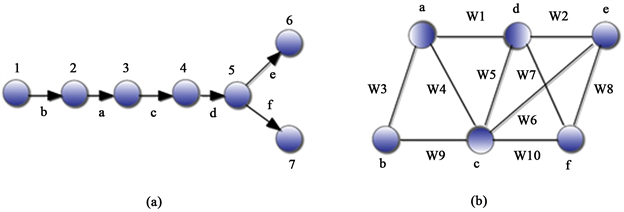Figure 1. An illustration of network topology. (a) Topology example; (b) Corresponding channel contention

2.3. 按比例公平分配资源模型

${P}_{j}$ 代表所有能够直接或者间接利用红色链路j牺牲的数据流来发送数据流的链路的集合，当链路 满足以下的条件时，即成为集合 ${P}_{j}$ 中的元素：

$\forall i\in {P}_{j},\left\{M\left(i\right)\subseteq M\left(j\right)\right\}||\left\{\left(M\left(i\right)\subseteq M\left(j\right)\right)\text{&}\left(M\left(i\right)\cap M\left(j\right)\ne \varnothing \right)\text{&}\left({C}_{1}||{C}_{2}\right)\right\}$

${C}_{1}\to \forall c\in \left\{M\left(i\right)\\M\left(j\right)\right\}:L\left(c\right)=\left\{i\right\}$

${C}_{2}\to \exists c\in \left\{M\left(i\right)\cap M\left(j\right)\right\}:\left(\exists k\in {P}_{j}&k\in L\left(c\right):\forall d\in M\left(k\right),L\left(d\right)\\\left\{i,j,k\right\}=\varnothing \right)$

${\text{rate}}_{k}=\mathrm{min}\left(\frac{{\text{min_resource}}_{1}}{p\left(k\right)},\text{min_resourc}{e}_{2}\right)$

$\forall c\in M\left(k\right),\text{resource}\left(c\right)=\text{resource}\left(c\right)-{\text{rate}}_{k}$

${\text{rate}}_{j}=\frac{{\text{resource}}_{c}}{\text{num_whitelinks}\left(c\right)},\text{\hspace{0.17em}}\text{\hspace{0.17em}}\text{where}\text{\hspace{0.17em}}c\in M\left(j\right)$

2.4. 贫穷节点与富裕节点

3. 分布式节点调度优化算法

1) 输入网络拓扑图G(V, E)，根据链路之间的竞争情况，将网络拓扑图G(V, E)转变成数据流竞争图G'(V', E', W)。

2) 通过数据流竞争图识别出所有的最大派别，将链路分成红色链路与白色链路。

3) 根据每一条链路所属最大派别的情况分配占用资源的比例。

4) 所有节点分为贫穷节点和富裕节点。

5) 节点调度过程：发送节点根据链路之间的干扰情况以及发送数据包大小选择接收节点传输数据流，但发送节点与接收节点不能是同一个节点。

4. 仿真结果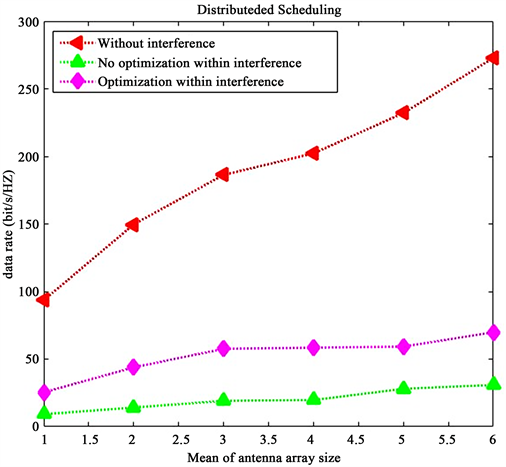Figure 2. Impact of mean of antenna array size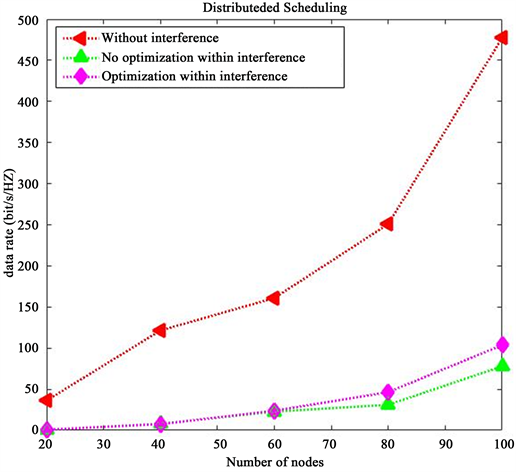Figure 3. Impact of node density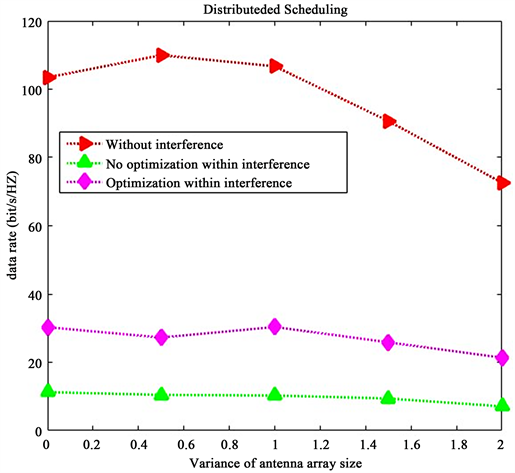Figure 4. Impact of variance of antenna array size

5. 结束语

Node Scheduling Optimization Algorithm in Heterogeneous Ad Hoc Networks Based on MIMO[J]. 无线通信, 2017, 07(05): 155-162. http://dx.doi.org/10.12677/HJWC.2017.75019

1. 1. Chu, S., Wang, X. and Yang, Y. (2014) Adaptive Scheduling in MIMO-Based Heterogeneous Ad Hoc Networks. IEEE Transactions on Mobile Computing, 13, 964-978. https://doi.org/10.1109/TMC.2013.112

2. 2. Nandagopal, T., Kim, T.E., Gao, X., et al. (2000) Achieving MAC Layer Fairness in Wireless Packet Networks. International Conference on Mobile Computing & Networking, Boston, 6-11August 2000, 87-98. https://doi.org/10.1145/345910.345925

3. 3. Sundaresan, K., Sivakumar, R., Ingram, M.A., et al. (2004) Medium Access Control in Ad Hoc Networks with MIMO Links: Optimization Considerations and Algorithms. IEEE Transactions on Mobile Computing, 3, 350-365. https://doi.org/10.1109/TMC.2004.42

4. 4. Tse, D. and Viswanath, P. (2009) Fundamentals of Wireless Communication. Posts and Telecom Press, Beijing, 1-5.

5. 5. Donald, J. and Tarjan, E.R. (1978) Algorithmic Aspects of Vertex Elimination. Siam Journal on Applied Mathematics, 34, 176-197. https://doi.org/10.1137/0134014

6. 6. Fulkerson, D.R. and Gross, O.A. (1965) Incidence Matrices and Interval Graphs. Pacific Journal of Mathematics, 15, 835-855. https://doi.org/10.2140/pjm.1965.15.835